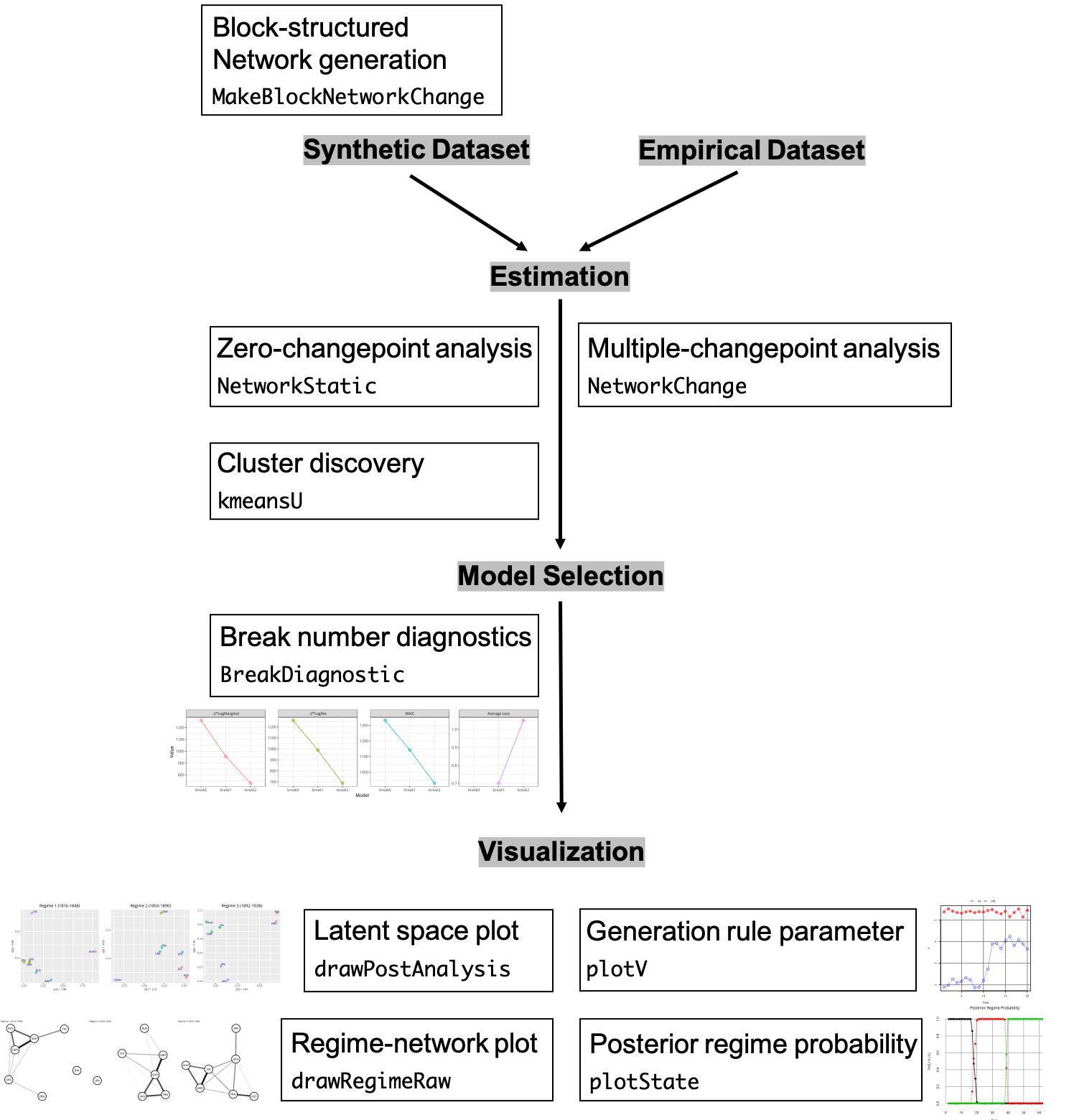# NetworkChange: Analyzing Network Changes in R

## Introduction

Many recorded network data span over a long period of time. Researchers often wish to detect “structural changes,” “turning points,” or “critical junctures” from these network data to answer substantively important questions. For example, the evolution of military alliance networks in international politics has been known to experience several breaks due to world wars and the end of Cold War. However, to this date, statistical methods to uncover network changes have been few and far between.

In this vignette, we explain how to use NetworkChange for network changepoint analysis. NetworkChange is an R package that detects structural changes in longitudinal network data using the latent space approach. Based on the Bayesian multi-array representation of longitudinal networks (Hoff 2011, 2015), NetworkChange performs Bayesian hidden Markov analysis (Chib 1998) to discover changes in structural network features across temporal layers. NetworkChange can handle various forms of network changes such as block-splitting, block-merging, and core-periphery changes. NetworkChange also provides functions for model diagnostics using WAIC, average loss, and log marginal likelihoods as well as visualization tools for dynamic analysis results of longitudinal networks.

library(NetworkChange)Summary of selected features and functions of the package.

## Input network data and synthetic data generation

Input data for NetworkChange takes an array form. Hence, the dimension of input data is $$N \times N \times T$$. Currently, NetworkChange allows longitudinal data of symmetric ($$i.e.$$ undirected) networks.

One quick way to generate a synthetic longitudinal network data set with a changepoint is to call MakeBlockNetworkChange(). It has three clusters by default and users can choose the size of data by selecting the number of nodes in each cluster ($$n$$) and the length of time ($$T$$). For example, if one chooses $$n=10$$ and $$T=20$$, an array of $$30 \times 30 \times 20$$ is generated. base.prob is the inter-cluster link probability, and block.prob+base.prob is the intra-cluster link probability. When one sets block.prob>0, a clustered network data set is generated. If block.prob=0, we have a random network data set.

set.seed(11173)
n <- 10 ## number of nodes in each cluster
Y <- MakeBlockNetworkChange(n=n, break.point = .5,
base.prob=.05, block.prob=.7,
T=20, type ="split")
dim(Y)
#>  30 30 20

The above code generates a longitudinal network data set with a changepoint in the middle (break.point=0.5). We specify the type of network change as a block-splitting change (type =“split”}) where the initial two cluster network splits into three clusters.

Currently, MakeBlockNetworkChange() provides five different options of network changes (type): “constant”, “merge”, “split”, “merge-split”, and “split-merge.” If “constant” is chosen, the number of breaks is zero. If “merge” or “split” is chosen, the number of breaks is one. If either “merge-split” or “split-merge” is chosen, the number of breaks is two.

Users can use plotnetarray() to visualize the longitudinal network data. The resulting plot shows a selected number of equi-distant time-specific networks. By default, n.graph is 4. Users can change plot settings by changing options in ggnet.

plotnetarray(Y)`